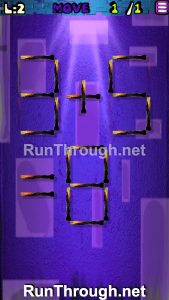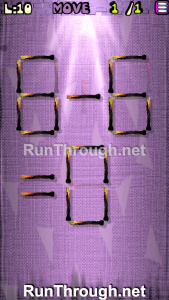# Move the Matches Puzzles Episode 9 Level 1-20 Walkthrough

Walkthrough for all the solutions and answers to the game Move the Matches Puzzles by K-Lab. Get the app on the Google Play Store to play along if you do not already have it. This is for episode 9, levels 1 through 20.

For the walkthrough (or answer) images, we will be showing the final image and answer of the Matches Puzzle. This will allow you to see where everything goes, and to know for sure that the puzzle works.  These puzzles have been recently updated, so other walkthroughs do not have the updated puzzles, but we try to always stay up to date with every walkthrough and answer!

### Level 1Instructions: Add 9 matches to get 6 triangles

### Level 2Instructions: Move 1 match to correct the equation

Solution: 3+5=8

### Level 3Instructions: Add 2 matches to correct the equation

Solution: 8/8=1

### Level 4Instructions: Add 3 matches to correct the equation

Solution: 7×7=49

### Level 5Instructions: Move 4 matches to get 3 squares

### Level 6Instructions: Move 1 match to correct the equation

Solution: 3×3=9

### Level 7Instructions: Move 1 match to correct the equation

Solution: 3+8=11

### Level 8Instructions: Remove 2 matches to correct the equation

Solution: 4×9=36

### Level 9Instructions: Move 1 match to correct the equation

Solution: 9-9=0

### Level 10Instructions: Move 1 match to correct the equation

Solution: 6-6=0

### Level 11Instructions: Move 3 matches to get 4 squares

### Level 12Instructions: Move 1 match to correct the equation

Solution: 6/6=1

### Level 13Instructions: Add 3 matches to correct the equation

Solution: 6×6=36

### Level 14Instructions: Add 3 matches to correct the equation

Solution: 8-2=6

### Level 15Instructions: Move 1 match to correct the equation

Solution: 7×7=49

### Level 16Instructions: Move 2 matches to correct the equation

Solution: 9×4=36

### Level 17Instructions: Move 2 matches to get 3 squares

### Level 18Instructions: Move 2 matches to correct the equation

Solution: 6×8=48

### Level 19Instructions: Move 1 match to correct the equation

Solution: 2×3=6

### Level 20Instructions: Remove 2 matches to correct the equation

Solution: 9/3=3